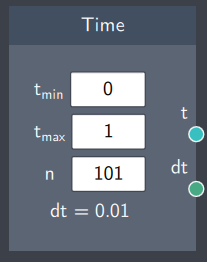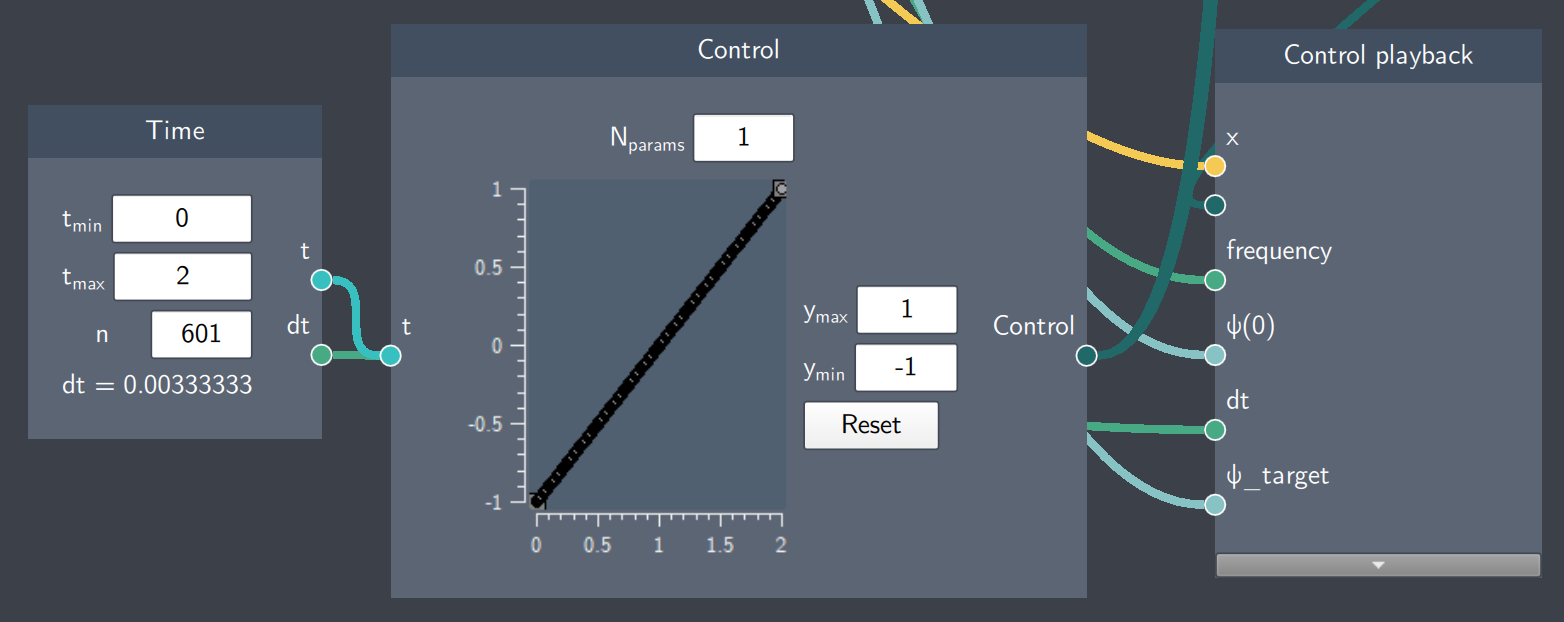# Time

## Description

The Time node defines the time axis. It represents the bounds and the time resolution for a control path.

Note that this node is not used for dynamical time evolution, which is performed by placing a Time Evolution node in a  For Loop.## Input

The node does not require any input from other nodes.

## Content

The Time node consists of  3 content fields that are used to generate the discrete time dimension. $t_{min}$ and $t_{max}$ represent the lower and upper bound of the time axis respectively and $n$ is the number of points within this range. It displays the timestep ($dt$) based on the content fields.

## Output

• Time (t): This output is $n$ values of $t$ within a specified range based on the content fields
• Timestep (dt): This output is a scalar value that defines the timestep

## Example

In the example below, the time axis has 0 and 2 as its lower and upper bound respectively. The number of steps in this range is n=601 and each timestep (dt) is 0.003. The output $t$ from the Time node is connected to the Control node where the x-axis denotes time and $dt$ is connected to a time loop.